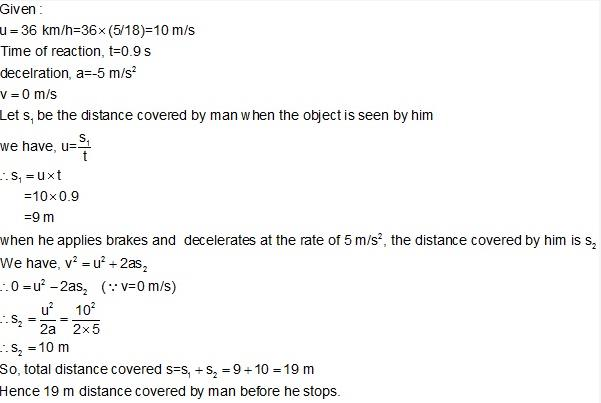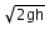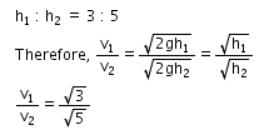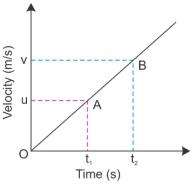Courses

# Test: Equations Of Motion

## 10 Questions MCQ Test Science Class 9 | Test: Equations Of Motion

Description
This mock test of Test: Equations Of Motion for Class 9 helps you for every Class 9 entrance exam. This contains 10 Multiple Choice Questions for Class 9 Test: Equations Of Motion (mcq) to study with solutions a complete question bank. The solved questions answers in this Test: Equations Of Motion quiz give you a good mix of easy questions and tough questions. Class 9 students definitely take this Test: Equations Of Motion exercise for a better result in the exam. You can find other Test: Equations Of Motion extra questions, long questions & short questions for Class 9 on EduRev as well by searching above.
QUESTION: 1

### A man is moving with 36 kmph. The time of reaction is 0.9 seconds. On seeing an obstacle in the path, he applies brakes and decelerates at 5 m/s2, the total distance covered before he stops is:

Solution:QUESTION: 2

### The ratio of the heights from which two bodies are dropped is 3:5 respectively. The ratio of their final velocities is:​

Solution:

Velocity acquired by the two bodies falling from rest through a distance his given as v =Given that :QUESTION: 3

### A body starts to slide over a horizontal surface with an initial velocity of 0.2 m/s. Due to friction, its velocity decreases at the rate of 0.02 m/s2. How much time will it take for the body to stop?

Solution:
Initial velocity, u = 0.2 m/s.
Final velocity, v = 0
Acceleration, a = 0.02 m/s^2.
v = u + at
0 = 0.2 - 0.02 x t
0.02 x t = 0.2
t = 0.2/0.02 = 10 sec.
Thus, the body will take 10 seconds to stop.
QUESTION: 4

If you whirl a stone on the end of the string and the string suddenly breaks, the stone will:

Solution:
The correct option is A because when a stone is whirling around ,tied to a string its direction of the motion is constantly changing ,even if the speed/velocity is uniform.At the time of the string breaking,the stone because of it inertia of motion follows the direction which it had at the time of string breaking,which is mostly the tangent.
QUESTION: 5

To describe the motion of an object we first specify a

Solution: Yes this is because we need to observe the motion of object or rest of object from any point called reference point.
QUESTION: 6

The third equation of motion is

Solution:

The third equation of motion is :-
( v² - u² = 2as)
Here,
u = initial velocity
v =  final velocity
a =  acceleration
s =  distance

QUESTION: 7

The time taken by a train to slow down from 80 kmh-1 to 20 kmh-1 with a uniform deceleration of 2 ms-2 is

Solution:

v= 80*1000/3600 = 22.22 u=20*1000/3600=5.55 , Now a = v-u/t = 22.22-5.55/2 = 8.335

QUESTION: 8

The equations of motion can be derived by using:

Solution:

Consider a velocity - time graph for a uniformly accelerated body starting from rest is represented as follows.u = velocity at time t1
v =  velocity at time t2
If acceleration is represented as a, then, acceleration is defined as the rate of change in velocity.
⇒  a = v-u / t2 - t1
⇒ a = v-u /t
Or,v-u = at
⇒ v= u + at

QUESTION: 9

A racing car has a uniform acceleration of 6 m/s2. In 10s it will cover:​

Solution:

Given:Acceleration = 6 m/s^2Time = 10 secAs car start from rest so intial velocity will be zero.u = 0S = ut + 1/2(at^2)S = 0 + 1/2 x 6 x 100S = 300 m.

QUESTION: 10

A body performs an accelerated motion, with uniform speed. The motion of body is

Solution: This is because in circular motion body is continuously changing its speed and acceleration is the change of velocity.